Next: Dynamical Collapse Up: Ambipolar Diffusion Previous: Ionization Rate   Contents

## Ambipolar Diffusion

The ionization fraction of the gas in the cloud is low. Neutral gas and the ions are coupled via mutual collisions and ions are coupled with the magnetic field. Thus, the neutral molecules, a major component of the gas, are coupled with the magnetic field indirectly via ionized ions. Equation of motion for the molecular gas is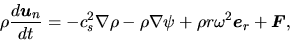(4.37)

where the forces appeared in the rhs represent, respectively, the pressure force, the self-gravity, the centrifugal force, and the force exerted on the neutral component per unit volume through two-body collision with ions. The friction force has a form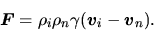(4.38)

We have assumed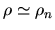since the mass density of the charged component is much smaller than that of the neutral one. On the other hand, that for ions is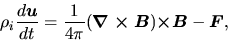(4.39)

where we ignored the pressure and self-gravity forces compared with the Lorentz force. If the inertia of the ions are ignored [lhs of eq.(4.39)=0], equations (4.37) and (4.39) give the equation of motion similar to that of the one-fluid as follows: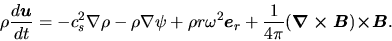(4.40)

Ignoring the inertia of the ions, equation (4.39) indicates that the friction force should be balanced with the Lorentz force as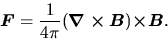(4.41)

Using equations (4.36) and (4.38), this gives the drift velocity of ions against the neutrals as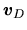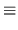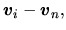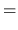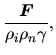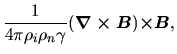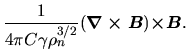(4.42)

Since the Lorentz force works outwardly, the drift velocityalso points outwardly. Viewing from the ions and magnetic field lines,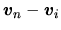points inwardly. Thus, the mass in a flux tube varies with time; that of the cloud center increases while that of the cloud surface decreases. The ambipolar diffusion is a process to change the mass distribution against the magnetic flux tube.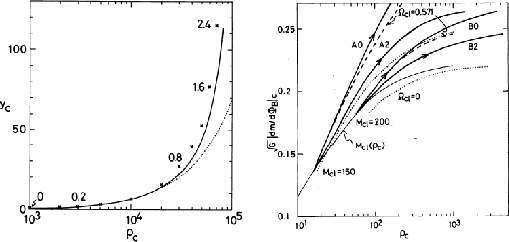How is the structure affected by the increase of mass/flux ratio at the cloud center? Here, we will derive the relation between the mass-to-flux ratio and central density and answer the question. Numerical calculation is necessary for the axisymmetric (realistic) cloud. However, this can be obtained for the case of a plane-parallel disk in which the magnetic field is running parallel to the disk, For the plane-parallel disk the hydrostatic balance is achieved as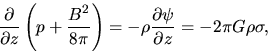(4.43)

where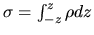. Equation (4.43) is integrated to obtain the relation between the variables at the center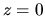as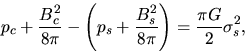(4.44)

where we used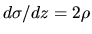. This is rewritten as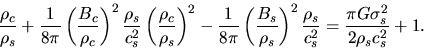(4.45)

In the second term of lhs,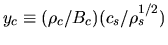is a nondimensional mass flux ratio at the center and this is related to the center-to-surface density ratio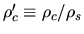. Using these nondimensional variables, equation (4.45) is expressed as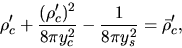(4.46)

where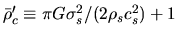represents the central density necessary for the disk to be supported without a magnetic field (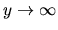). Figure 4.4 (left) illustrates this relation. This shows that if the central mass-to-flux ratio increases (moving upward), the central density increases. In low density,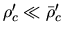, equation (4.46) indicates that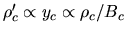. This means that on the low density regime, the density increases but the strength of magnetic field does not increase. Equation (4.46) also indicates that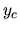increase much as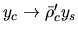when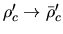. This relation between mass-to-flux ratio and the central density is well fitted to the numerical calculation of plane-parallel disk cloud driven by the ambipolar diffusion by Mouschovias, Paleologou, & Fiedler (1985). Does this indicate that the mass-to-flux ratio increases much when the central density increases, for example, from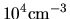to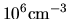?

From this point, quasistatic evolution of the magnetized cloud was first studied by Nakano (1979) adopting a method seeking for magnetohydrostatic configuration (Mouschovias 1976a, 1976b). He obtained that the magnetic flux to mass ratio near the center of the cloud does not decrease much below a critical density above which magnetohydrostatic configurations no more exist. Paleologou & Mouschovias (1983), Shu (1983), and Mouschovias, Paleologou, & Fiedler (1985) gave a completely different result. That is, the magnetic flux to mass ratio near the center decreases much when the density becomes larger than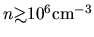. Critical discussion has been done between them4.1. However, we have now realized that the difference comes from the geometry assumed.

Relation of the mass-to-flux ratio to the central density for the axisymmetric realistic (not plane-parallel disk) cloud is plotted in Figure 4.4 (right) (Tomisaka, Ikeuchi, & Nakamura 1990). The central mass-to-flux ratio increases by the ambipolar diffusion. This increase the central density. Lines with arrows indicate the evolutionary paths driven by the ambipolar diffusion. It is to be noticed that the relation is completely different from that of the plane-parallel disk. Increase of the mass-flux ratio is small although the central density increases much. This indicates that in the quasistatic evolution driven by the ambipolar diffusion the mass-flux ratio does not increase much in contrast to the plane-parallel case.

In fully ionized plasma, the magnetic field is coupled to the matter. However, in the low ionized gas the magnetic field is coupled only to the ions. Thus the induction equation of magnatic field should be written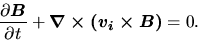(4.47)

This leads to the final expression as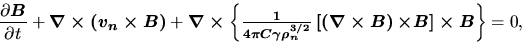(4.48)

where we used the expression of the drift velocity [equation (4.42)]. In the isothermal cloud, there is a maximum mass supported by the magnetic field. Since in the subcritical cloud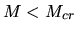the magnetic flux escapes from the center, the critical mass decreases with time. After the critical mass becomes smaller than the actual mass (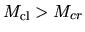), there is no hydrostatic configuration. The cloud evolves into the supercritical cloud region and experiences dynamical collapse.Next: Dynamical Collapse Up: Ambipolar Diffusion Previous: Ionization Rate   Contents
Kohji Tomisaka 2007-07-08